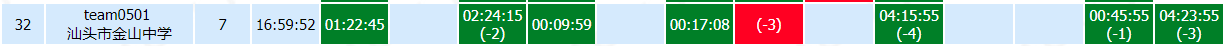2018 Multi-University Training Contest 3 比赛记录Sakits 7月 30, 2018# A. Ascending Rating

### solved by Sakits. 01:22:45(+)

直接倒着单调队列就好了，最值为队头，个数为队列长度。

# C. Dynamic Graph Matching

### solved by reek. 02:24:15(+2)

胡爷爷直接记忆化搜索过去了，因为匹配数其实不多，其实感觉就是状压DP。。。
跟边的加入顺序无关，所以可以设$f[S]$表示点被选择的情况为$S$的方案数，加一条边$x-y$，状态从大到小更新$f[S]+=f[S-x-y]$，删一条边，状态从大到小更新$f[S]-=f[S-x-y]$即可。

# D. Euler Function

### solved by reek. 00:09:59(+)

随便画画很容易发现只有$1,2,3,4,6$的欧拉函数是质数。
证明很简单，欧拉公式的式子一写看看前面几个质数的系数就好了。

# F. Grab The Tree

### solved by reek. 00:17:08(+)

考虑高位到低位贪心，如果这一位为$1$的个数为偶数，选不选并不会影响答案，如果为奇数，那么只选一个数就必胜了。
所以如果有一位为$1$的数个数为奇数，那么先手必胜，否则平局。

# I. Random Sequence

### solved by reek. 04:15:55(+4)

果然复杂度都是假的。。。跑满了肯定T，结果居然跑过去了。。。
设$f[i][j][k][l]$表示决策第$i$个数，前$3,2,1$个数的$gcd$分别为$j,k,l$的期望。
显然满足$j|k, k|l$，后三维的状态数最大为$1471$，转移$O(m)$，复杂度$O(nmS)$，这居然跑过去了，又被安排了…

算几个坐标，按题意模拟即可。

# M. Walking Plan

### solved by Sakits. 04:23:55(+3)

平衡一下各部分复杂度就能跑过去了。
处理询问的时候枚举中间点，二进制下前$7$位和后$7$位分开来得到答案。
预处理$f[i][j][k]$表示跑恰好$i\times 2^7$步，$j$$k$的最短路，$g[i][j][k]$表示跑至少$i$步，$j$$k$的最短路就行了。